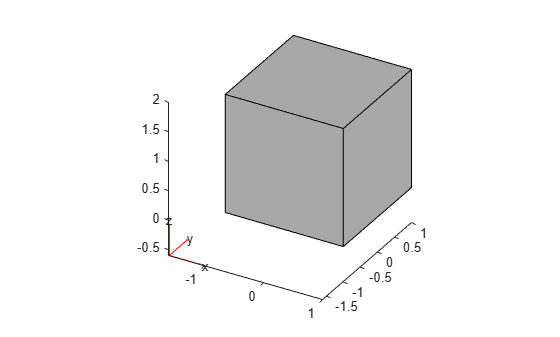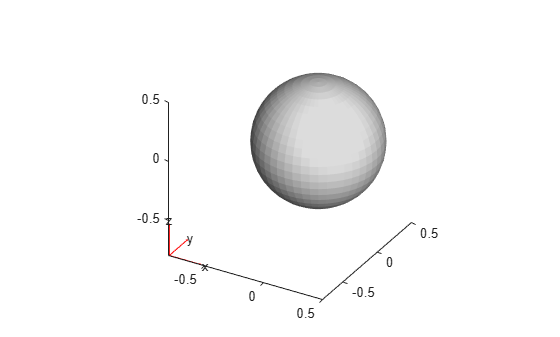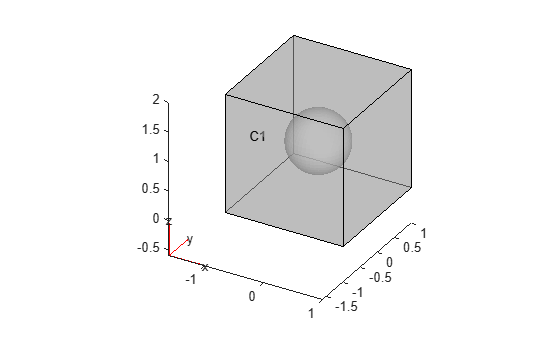# Sphere in Cube

This example shows how to create a nested multidomain geometry consisting of a unit sphere and a cube. The first part of the example creates a cube with a spherical cavity by using `alphaShape`. The second part creates a solid sphere using tetrahedral elements, and then combines all tetrahedral elements to obtain a solid sphere embedded in a cube.

### Cube with Spherical Cavity

First, create a geometry consisting of a cube with a spherical cavity. This geometry has one cell.

Create a 3-D rectangular mesh grid.

```[xg, yg, zg] = meshgrid(-2:0.25:2); Pcube = [xg(:) yg(:), zg(:)];```

Extract the grid points located outside of the unit spherical region.

`Pcavitycube = Pcube(vecnorm(Pcube') > 1,:);`

Create points on the unit sphere.

```[x1,y1,z1] = sphere(24); Psphere = [x1(:) y1(:) z1(:)]; Psphere = unique(Psphere,'rows');```

Combine the coordinates of the rectangular grid (without the points inside the sphere) and the surface coordinates of the unit sphere.

`Pcombined = [Pcavitycube;Psphere];`

Create an `alphaShape` object representing the cube with the spherical cavity.

```shpCubeWithSphericalCavity = alphaShape(Pcombined(:,1), ... Pcombined(:,2), ... Pcombined(:,3)); figure plot(shpCubeWithSphericalCavity,'FaceAlpha',0.4) title('alphaShape: Cube with Spherical Cavity')```Recover the triangulation that defines the domain of the `alphaShape` object.

`[tri,loc] = alphaTriangulation(shpCubeWithSphericalCavity);`

Create a PDE model.

`modelCube = createpde;`

Create a geometry from the mesh and import the geometry and the mesh into the model.

`[gCube,mshCube] = geometryFromMesh(modelCube,loc',tri');`

Plot the resulting geometry.

```figure pdegplot(modelCube,'FaceAlpha',0.5,'CellLabels','on') title('PDEModel: Cube with Spherical Cavity')```### Solid Sphere Nested in Cube

Create tetrahedral elements to form a solid sphere by using the spherical shell and adding a new node at the center. First, obtain the spherical shell by extracting facets of the spherical boundary.

```sphereFacets = boundaryFacets(mshCube,'Face',3); sphereNodes = findNodes(mshCube,'region','Face',3);```

Add a new node at the center.

`newNodeID = size(mshCube.Nodes,2) + 1;`

Construct the tetrahedral elements by using each of the three nodes on the spherical boundary facets and the new node at the origin.

`sphereTets = [sphereFacets; newNodeID*ones(1,size(sphereFacets,2))];`

Create a model that combines the cube with the spherical cavity and a sphere.

`model = createpde;`

Create a vector that maps all `mshCube` elements to cell 1, and all elements of the solid sphere to cell 2.

`e2c = [ones(1,size(mshCube.Elements,2)), 2*ones(1,size(sphereTets,2))];`

Add a new node at the center `[0;0;0]` to the nodes of the cube with the cavity.

`combinedNodes = [mshCube.Nodes,[0;0;0]];`

Combine the element connectivity matrices.

`combinedElements = [mshCube.Elements,sphereTets];`

Create a two-cell geometry from the mesh.

```[g,msh] = geometryFromMesh(model,combinedNodes,combinedElements,e2c); figure pdegplot(model,'FaceAlpha',0.5,'CellLabels','on') title('Solid Sphere in Cube')```##### SupportGet trial now